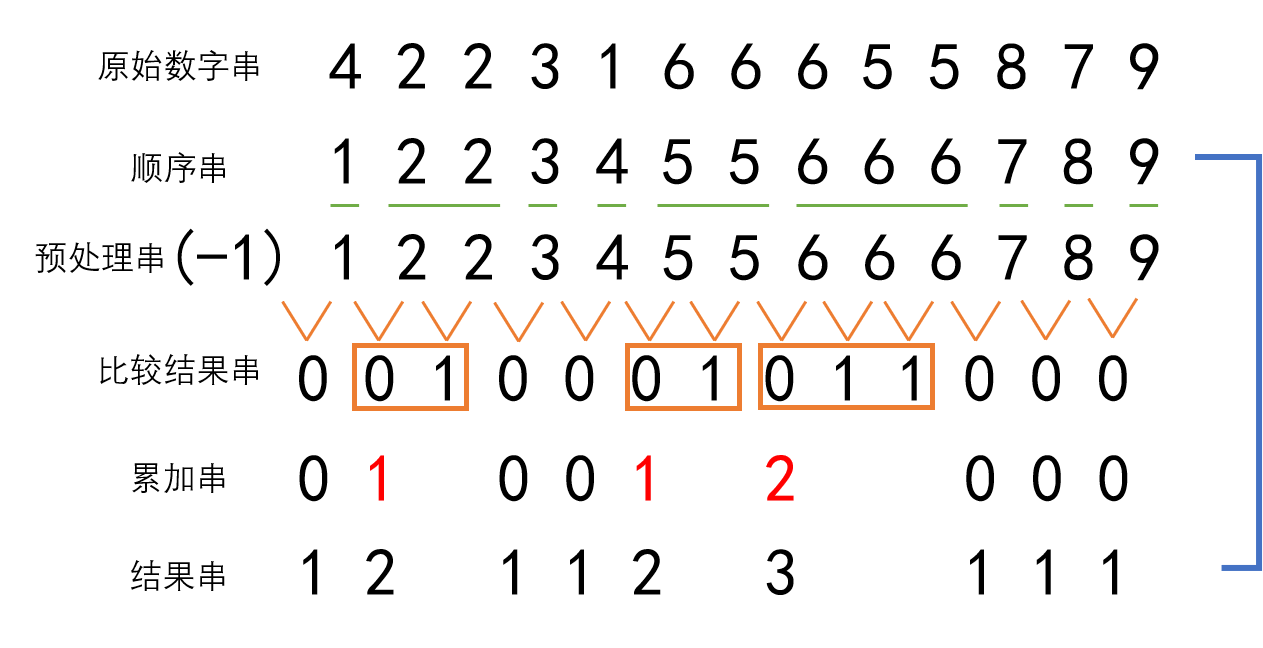# 图像二维熵计算的C++实现

## Aug 27,2019   6433 words   23 min

#### 1.自定义数据结构体

``````struct IJpair {
// 自定义IJpair数据格式用于存放数值对
// 同时重载了==和<使其可以进行大小比较

int center_p;
int mean_p;

bool operator==(const IJpair &ij) const {
return center_p == ij.center_p && mean_p == ij.mean_p;
}

// 比较大小的规则是center_p小的在前，center_p相同时mean_p小的在前
bool operator<(const IJpair &ij) const {
if (center_p < ij.center_p) {
return true;
} else if (center_p == ij.center_p) {
if (mean_p < ij.mean_p) {
return true;
} else {
return false;
}
} else {
return false;
}
}
};
``````

#### 2.重复元素个数统计

##### (1)常规方法

``````#include <iostream>
#include <algorithm>

using namespace std;

struct IJpair {
// 自定义IJpair数据格式用于存放数值对
// 同时重载了==和<使其可以进行大小比较

int center_p;
int mean_p;

bool operator==(const IJpair &ij) const {
return center_p == ij.center_p && mean_p == ij.mean_p;
}

// 比较大小的规则是center_p小的在前，center_p相同时mean_p小的在前，如(1,2)<(1,3)<(2,1)
bool operator<(const IJpair &ij) const {
if (center_p < ij.center_p) {
return true;
} else if (center_p == ij.center_p) {
if (mean_p < ij.mean_p) {
return true;
} else {
return false;
}
} else {
return false;
}
}
};

int main() {
vector<IJpair> IJs;
IJs.push_back(IJpair{5, 2});
IJs.push_back(IJpair{3, 2});
IJs.push_back(IJpair{5, 2});
IJs.push_back(IJpair{7, 6});
IJs.push_back(IJpair{3, 2});
IJs.push_back(IJpair{6, 4});
IJs.push_back(IJpair{1, 3});
IJs.push_back(IJpair{5, 2});
IJs.push_back(IJpair{1, 3});
IJs.push_back(IJpair{2, 9});

// 首先得到一个不含重复元素的vector - IJ_set
vector<IJpair> IJ_set = IJs;
sort(IJ_set.begin(), IJ_set.end());
IJ_set.erase(unique(IJ_set.begin(), IJ_set.end()), IJ_set.end());

// 然后将IJ_set中的元素依次在IJs中遍历并统计个数
vector<int> item_num;
for (int l = 0; l < IJ_set.size(); ++l) {
int num = 0;
num = count(IJs.begin(), IJs.end(), IJ_set[l]);
item_num.push_back(num);
}

// 输出展示
for (int i = 0; i < item_num.size(); ++i) {
printf("(%d,%d) %d\n", IJ_set[i].center_p, IJ_set[i].mean_p, item_num[i]);
}
return 0;
}
``````

• `sort()`:用于对STL容器中的元素进行排序，常规使用格式是`sort(vec.begin(),vec.end())`，默认是从小到大排序。如果是自定义数据类型排序，记得重载`==``<`运算符。

• `unique()`:用于去除STL容器中的相邻的重复元素，用法是`unique(vec.begin(),vec.end())`，它会把重复的元素添加到容器末尾(所以容器大小并没有改变)，返回值是去重之后的尾地址。需要注意的是要想用它去重记得先对容器元素排序，使相同元素相邻。

• `erase()`:用于擦除内存，有三种调用方式：`earse(pos,n)`表示从pos开始擦除，擦除n个字符；`earse(pos)`表示擦除pos后的所有内容；`earse(first,last)`表示擦除first迭代器和last迭代器之间的内容。在上面的代码中便配合`unique()`函数实现了去除重复元素。

• `count()`:用于统计容器中指定元素的出现次数，用法是`count(vec.begin(),vec.end(),item)`。与之类似的还有`count_if()`函数，顾名思义是统计容易中满足某个条件的元素个数。

##### (2)自己的方法

``````#include <iostream>
#include <algorithm>

using namespace std;

struct IJpair {
// 自定义IJpair数据格式用于存放数值对
// 同时重载了==和<使其可以进行大小比较

int center_p;
int mean_p;

bool operator==(const IJpair &ij) const {
return center_p == ij.center_p && mean_p == ij.mean_p;
}

// 比较大小的规则是center_p小的在前，center_p相同时mean_p小的在前，如{1,2}<{1,3}<{2,1}
bool operator<(const IJpair &ij) const {
if (center_p < ij.center_p) {
return true;
} else if (center_p == ij.center_p) {
if (mean_p < ij.mean_p) {
return true;
} else {
return false;
}
} else {
return false;
}
}
};

int main() {
vector<IJpair> IJs;
IJs.push_back(IJpair{5, 2});
IJs.push_back(IJpair{3, 2});
IJs.push_back(IJpair{5, 2});
IJs.push_back(IJpair{7, 6});
IJs.push_back(IJpair{3, 2});
IJs.push_back(IJpair{6, 4});
IJs.push_back(IJpair{1, 3});
IJs.push_back(IJpair{5, 2});
IJs.push_back(IJpair{1, 3});
IJs.push_back(IJpair{2, 9});

// 第一步，相邻元素两两比较得到结果串
sort(IJs.begin(), IJs.end());
vector<int> times;
times.push_back(0);
for (int i = 0; i < IJs.size() - 1; ++i) {
if (IJs[i] == IJs[i + 1]) {
times.push_back(1);
} else {
times.push_back(0);
}
}

// 第二步，统计结果串中非零元素个数并求和
vector<int> times2;
int sum = 0;
for (int j = 0; j < times.size() - 1; ++j) {
if (times[j] == 0 and times[j + 1] == 0) {
times2.push_back(times[j] + 1);
} else if (times[j] == 1 and times[j + 1] == 0) {
times2.push_back(sum + 1);
sum = 0;
} else {
sum += 1;
if (j == times.size() - 2) {
times2.push_back(sum + 1);
}
}
}
if (times[-1] == 0) {
times2.push_back(1);
}

// 得到不重复元素列表用于显示
vector<IJpair> IJ_set = IJs;
sort(IJ_set.begin(), IJ_set.end());
IJ_set.erase(unique(IJ_set.begin(), IJ_set.end()), IJ_set.end());
for (int i = 0; i < times2.size(); ++i) {
printf("(%d,%d) %d\n", IJ_set[i].center_p, IJ_set[i].mean_p, times2[i]);
}
return 0;
}
``````

###### 原理#### 4.参考资料

• https://www.cnblogs.com/SZxiaochun/p/7732250.html
• https://blog.csdn.net/u010141928/article/details/78671603
• https://blog.csdn.net/doctor_feng/article/details/11854485
• https://www.cnblogs.com/viviancc/p/4135712.html